To prove relation reflexive, transitive, symmetric and equivalent

Chapter 1 Class 12 Relation and Functions
Concept wise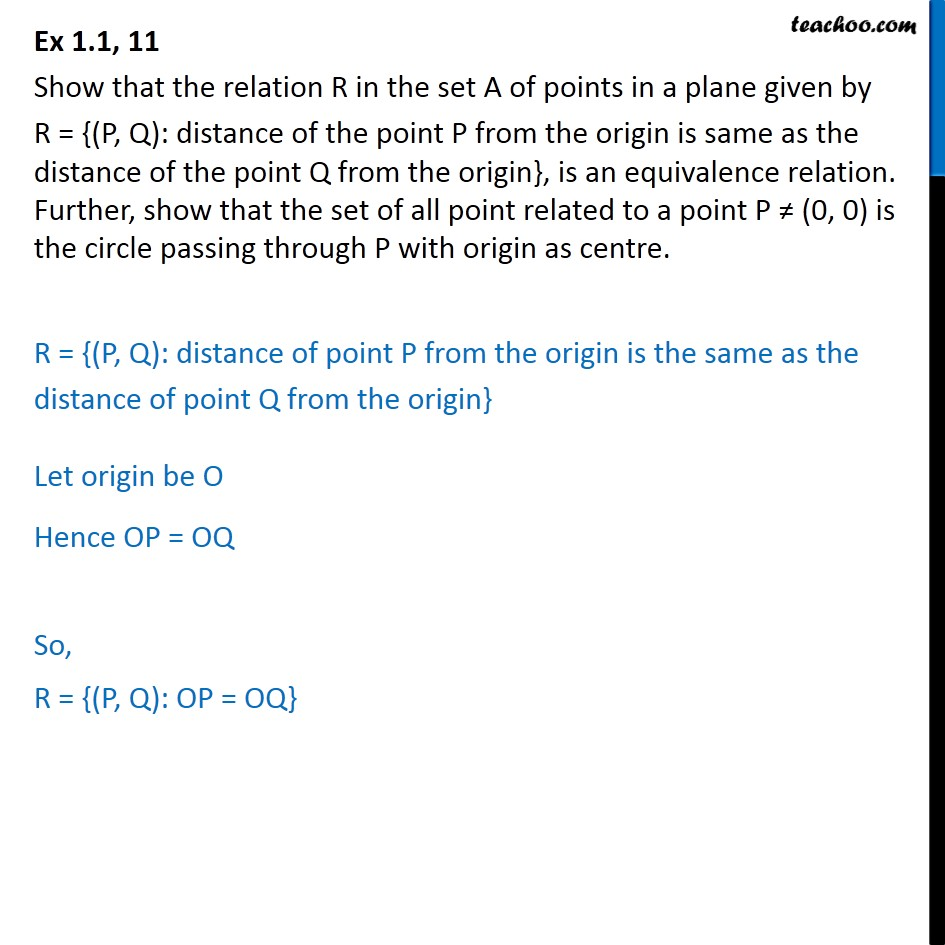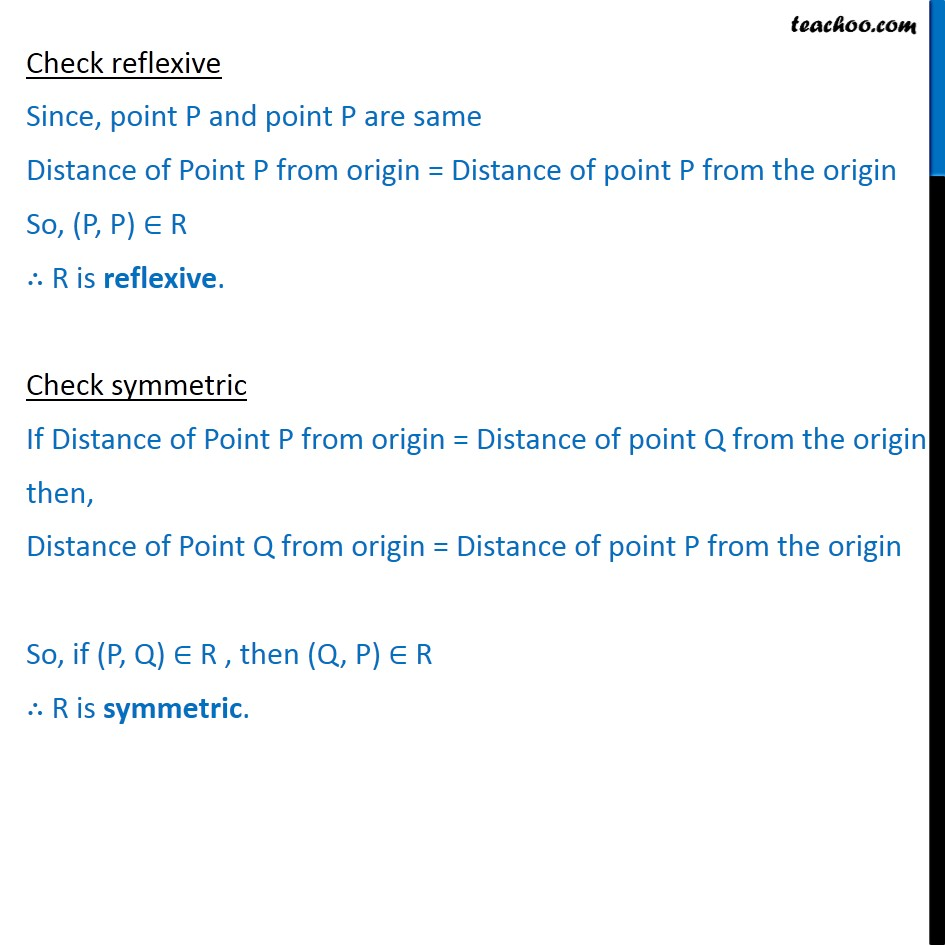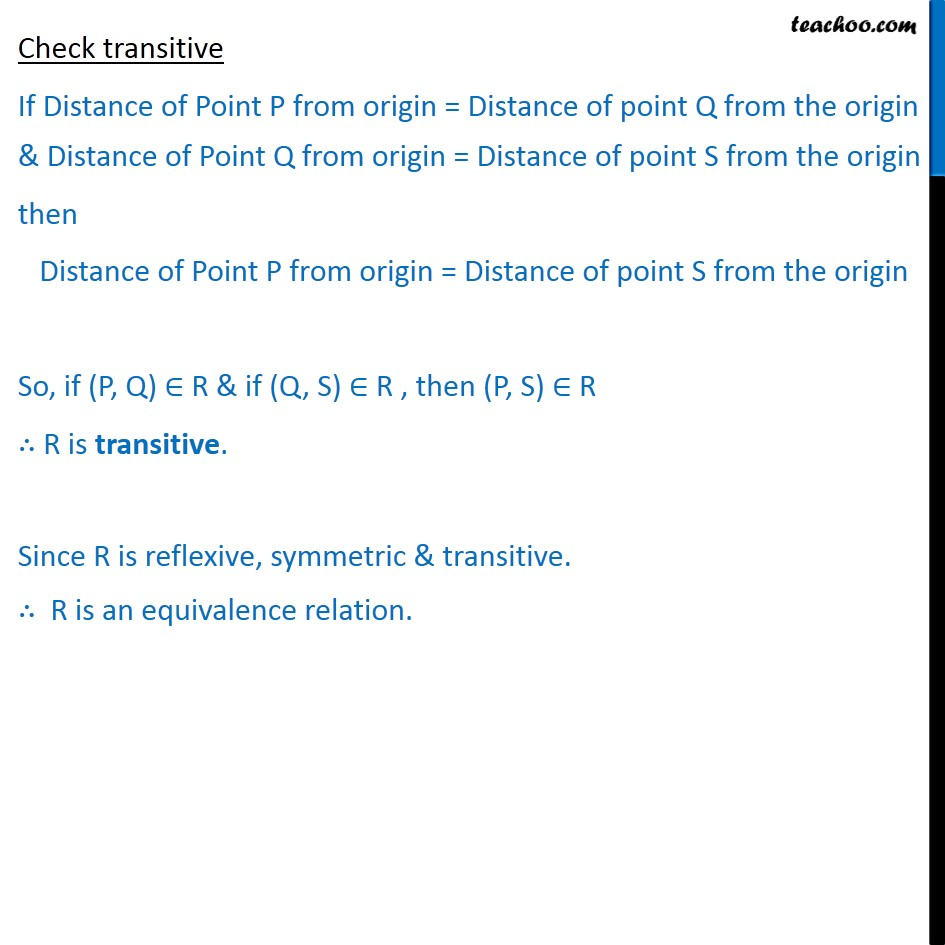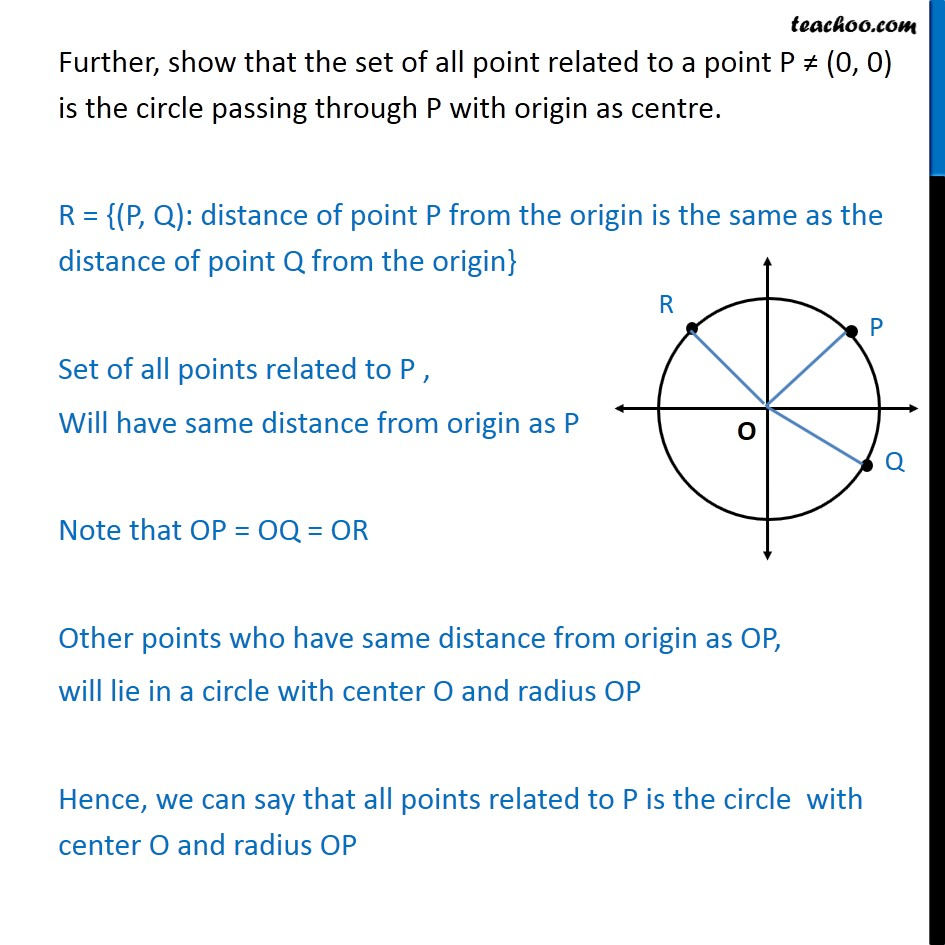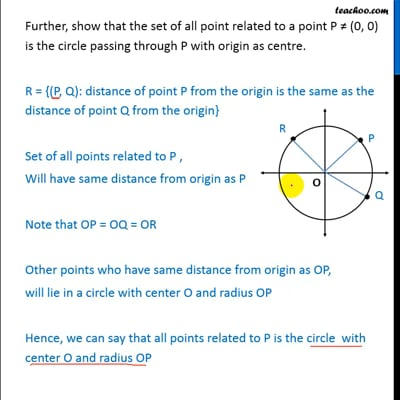This video is only available for Teachoo black users

Introducing your new favourite teacher - Teachoo Black, at only ₹83 per month

### Transcript

Ex 1.1, 11 Show that the relation R in the set A of points in a plane given by R = {(P, Q): distance of the point P from the origin is same as the distance of the point Q from the origin}, is an equivalence relation. Further, show that the set of all point related to a point P ≠ (0, 0) is the circle passing through P with origin as centre. R = {(P, Q): distance of point P from the origin is the same as the distance of point Q from the origin} Let origin be O Hence OP = OQ So, R = {(P, Q): OP = OQ} Check reflexive Since, point P and point P are same Distance of Point P from origin = Distance of point P from the origin So, (P, P) ∈ R ∴ R is reflexive. Check symmetric If Distance of Point P from origin = Distance of point Q from the origin then, Distance of Point Q from origin = Distance of point P from the origin So, if (P, Q) ∈ R , then (Q, P) ∈ R ∴ R is symmetric. Check transitive If Distance of Point P from origin = Distance of point Q from the origin & Distance of Point Q from origin = Distance of point S from the origin then Distance of Point P from origin = Distance of point S from the origin So, if (P, Q) ∈ R & if (Q, S) ∈ R , then (P, S) ∈ R ∴ R is transitive. Since R is reflexive, symmetric & transitive. ∴ R is an equivalence relation. Further, show that the set of all point related to a point P ≠ (0, 0) is the circle passing through P with origin as centre. R = {(P, Q): distance of point P from the origin is the same as the distance of point Q from the origin} Set of all points related to P , Will have same distance from origin as P Note that OP = OQ = OR Other points who have same distance from origin as OP, will lie in a circle with center O and radius OP Hence, we can say that all points related to P is the circle with center O and radius OP